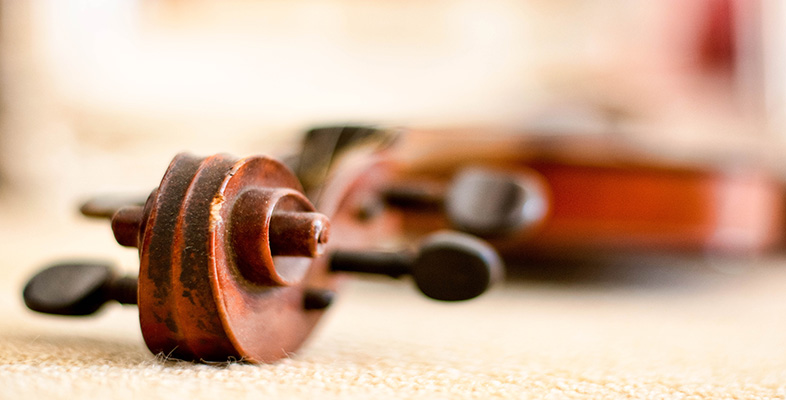Creating musical sounds

This free course is available to start right now. Review the full course description and key learning outcomes and create an account and enrol if you want a free statement of participation.

Free course

# 5.4 Vibrating string: normal modes of vibration

The frequencies at which standing waves can be set up on a string are the string's natural frequencies. They can be determined quite easily. The first thing to note is that the end of the string being held by the person is tightly gripped so any pulse or wave that returns to the person's hand will be reflected and inverted. Therefore both ends of the string can be considered to be fixed and so must be at nodes of the standing wave. But you learned earlier that the distance between adjacent nodes is half a wavelength. So, the length of the string must be an exact number of half wavelengths. That is, the string must be one half wavelength long, two half wavelengths long (i.e. one whole wavelength long), three half wavelengths long, four half wavelengths long (i.e. two whole wavelengths long), etc. If the length of the string is denoted by L and the wavelength by λ, then this can be expressed in the following way:This can be written more concisely as:where n is an integer number (i.e. 1, 2, 3, 4, … ).

Rearranging this equation, we can show that the wavelengths of standing waves that can be set up on the string are therefore given by:However, the frequency of a wave is related to its wavelength by the expression f = v/λ, where v is the wave speed. Therefore, the natural frequencies for the string are given by:The different standing-wave patterns, known as normal modes of vibration, are shown in Figure 10. The solid and dashed lines indicate the positions of the string at opposite phase positions in the cycle. You should be able to see that for each normal mode the string contains an integer number of half wavelengths.Figure 10 The first four normal modes of vibration of a string fixed at each end. The solid and dashed lines indicate the positions of the string at opposite phase positions in the cycle

In the first mode (n = 1) there are nodes at either end of the string but no nodes elsewhere on the string. The frequency at which this standing-wave pattern will be set up is f = v/2L. The first mode is known as the fundamental mode and, for this reason, the first natural frequency tends to be referred to as the fundamental frequency.

In the second mode (n = 2) there are again nodes at either end of the string but now there is also a node in the middle of the string. The frequency at which this standing-wave pattern will be set up is f = v/L. This is twice the value of the fundamental frequency.

In the third mode (n = 3) there are nodes at either end of the string and two more nodes positioned along the string. The frequency at which this standing-wave pattern will be set up is f = 3v/2L. This is three times the value of the fundamental frequency.

In the fourth mode (n = 4) there are the anticipated nodes at either end of the string and three more positioned at equal distances along the string. The frequency at which this standing-wave pattern will be set up is f = 2v/L. This is four times the value of the fundamental frequency.

You may well be seeing a trend emerging here! The frequencies at which standing waves are set up on the string are harmonically related. If the frequency at which the first mode occurs is denoted f1, then the frequencies at which the second, third and fourth modes occur are 2f1, 3f1 and 4f1 respectively. This set of frequencies and its indefinite continuation (5f1, 6f1, 7f1 … ) is known as a harmonic series. The fact that its natural frequencies form a harmonic series makes the vibrating string one of the most useful means of producing musical sounds.

## Activity 14

Calculate the first four natural frequencies of a 0.5 m long string that is fixed at each end. Assume the wave speed is 250 m/s.

First natural frequency f1 = v/2L = 250/(2 × 0.5) = 250 Hz.

Second natural frequency f2 = 2v/2L = (2 × 250)/(2 × 0.5) = 500 Hz.

Third natural frequency f3 = 3v/2L = (3 × 250)/(2 × 0.5) = 750 Hz.

Fourth natural frequency f4 = 4v/2L = (4 × 250)/(2 × 0.5) = 1000 Hz.

TA212_2